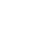معالجة البيانات المفقودة على التوزيع غير المتماثل
The problem of imputation of missing observations emerges in many areas. Data usually contained missing observations due to many factors, such as machine failures and human error. Incomplete dataset usually causes bias due to differences between observed and unobserved data. This paper proposed Neyman allocation method to estimate asymmetric winsorizing mean for handling missing observations when the data follow the exponential distribution. Different values of the exponential distribution parameters were used to illustrate. A set of data from exponential distribution were generated to compare the performance of the proposed methods such as regression trend, average of the whole data, naive forecast and average bound of the holes and the proposed Neyman allocation method. The goodness-of-fit criterions used were the mean absolute error (MAE) and the mean squared error (MSE). It was found that the proposed method gave the best fit in the sense of having smaller error, in particular for a large percentage of missing observations.
سنة النشـــر
2013